# Change Nickname

Current Nickname:
Search
v2.x
v2.x

# Degree | Centrality

## Degree

In graph theory, the degree of a vertex (node) of a graph is the number of edges that are incident to the node. In Ultipa Graph, all per-node degree operations are conducted in a pure real-time fashion. Only whole-graph degree operations are invoked as tasks (asynchronously) due to computational complexity, especially on large graphs.

`Note that in multigraphs, a loop (an edge whose starting node and ending node are identical) is counted as two edges`

### `algo(out_degree)`

Basic     Real-time

Out Degree is a per-node degree operation that calculates the total number of edges pointing away from a node, weight factor of edge could be applied.

Configuration items for out degree operations:

Item Data Type Specification Description
`<node_id>` int Ultipa ID To calculate for a specific node
`<edge_property_name>` string Edge property (numeric type) (Optional) The edge property (must be LTE first) to be used as the weight factor of edge, or use 1 as weight factor if not configured

Validity of `write_back()`:

Not supported.

Example 1: Calculate the Out Degree of node (_id = 12)

``````algo(out_degree)
.params( {node_id: 12} )
``````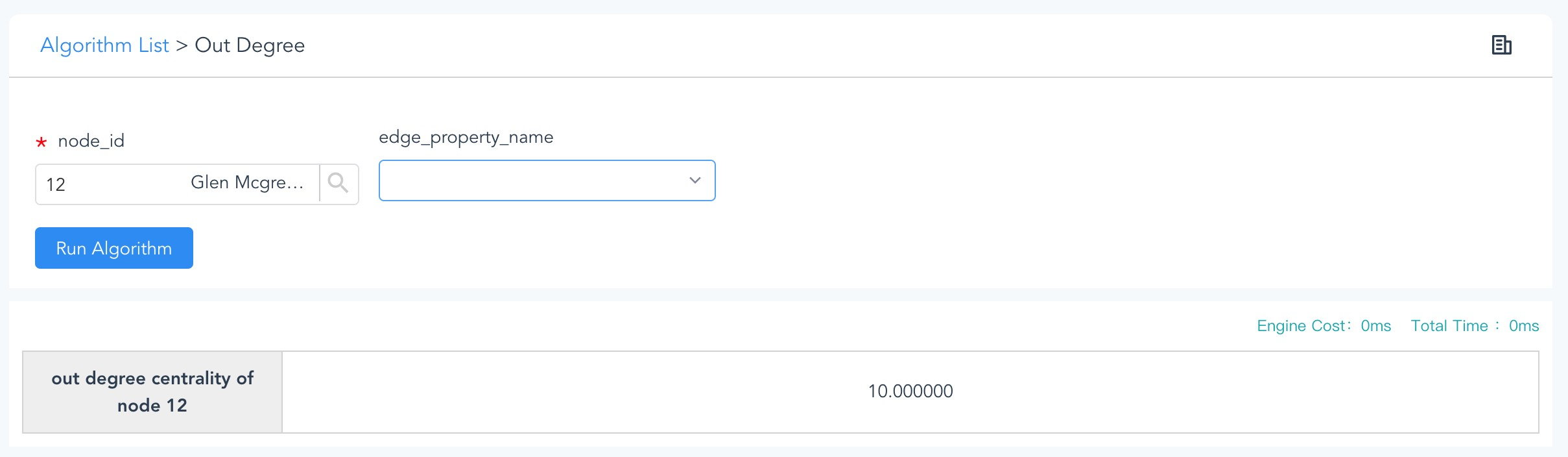Figure: Running real-time Algorithm --- Out Degree

Example 2: To verify the result of Example 1, spread from node (_id = 12) via right-directed edges.

``````spread()
.src(12).depth(1)
.direction(right).limit(1000)
``````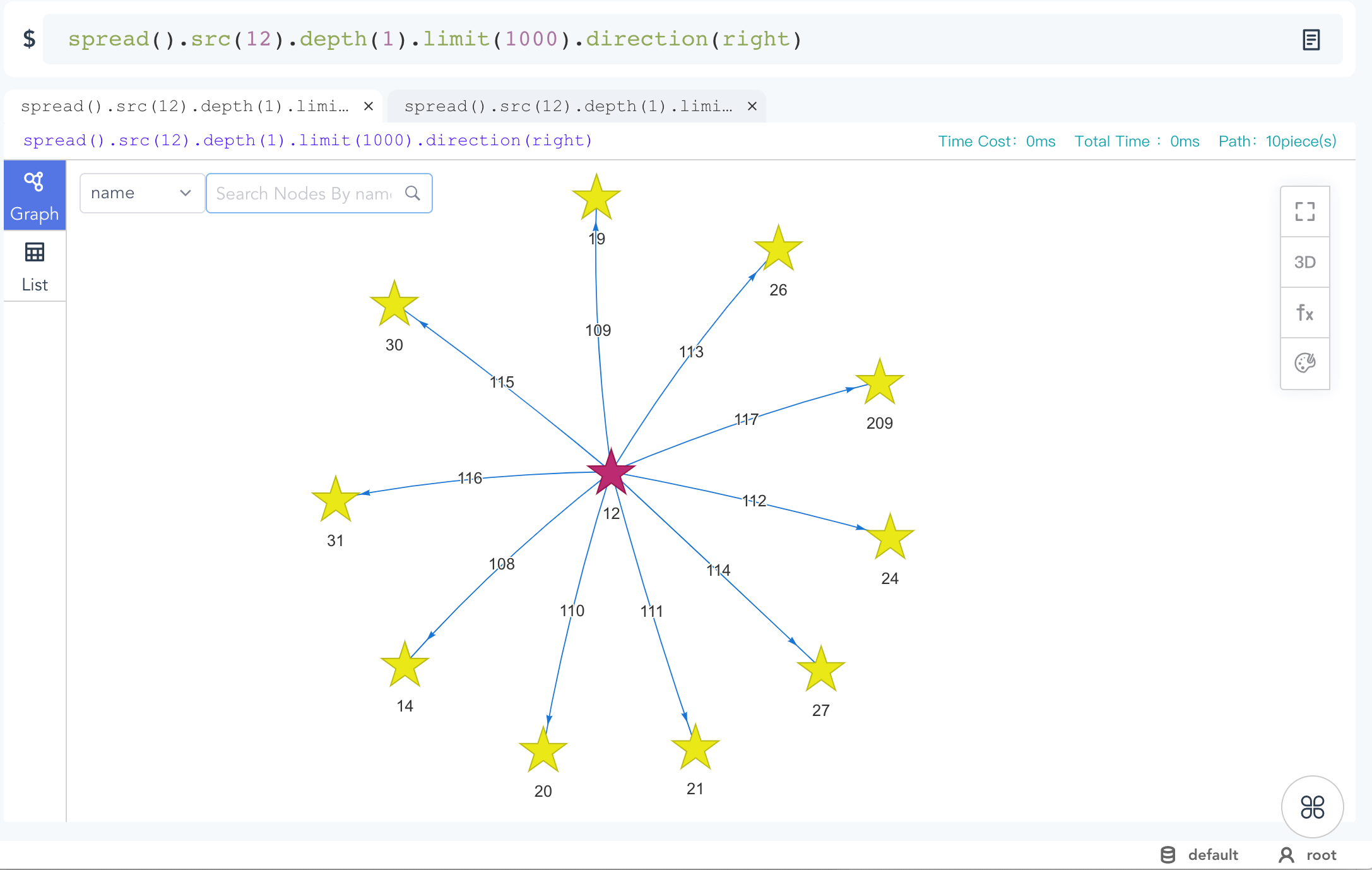Figure: Verifying Algorithm Calculation Results with spread()

Note: A `khop()` query is NOT a proper way to verify a degree operation, since that `khop()` query returns a bunch of 'de-duplicated' neighbors that do not reflect the number of incident edges.

Example 3: Same as Example 1, but using edge property 'rank' as weight factor, return the sum of all edges' rank values

``````algo(out_degree)
.params( {node_id: 12, edge_property_name: "rank"} )
``````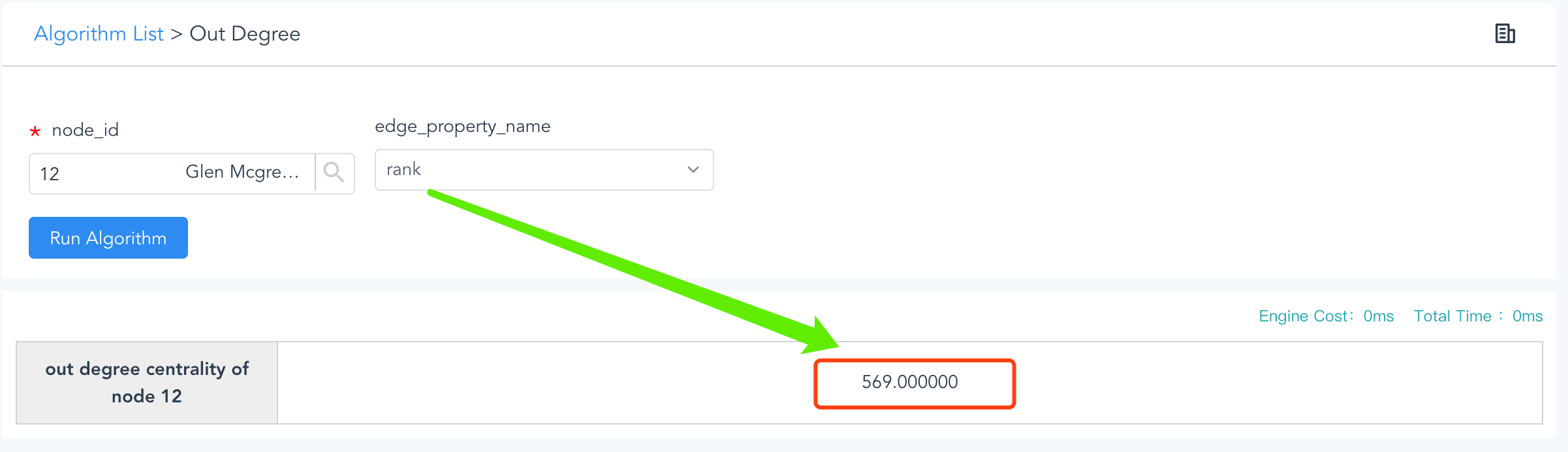Figure: Out Degree by Edge Property

The result shows that the Out Degree weighted by property 'rank' is 569 instead of 10 for a weightless Out Degree of node id=12.

### `algo(in_degree)`

Basic     Real-time

In Degree is comparable to Out Degree, but with calculations done against the inbound edges of node.

Configuration items for in degree operations:

Item Data Type Specification Description
`<node_id>` int Ultipa ID To calculate for a specific node
`<edge_property_name>` string Edge property (numeric type) (Optional) The edge property (must be LTE first) to be used as the weight factor of edge, or use 1 as weight factor if not configured

Validity of `write_back()`:

Not supported.

Example 1: Calculate the In Degree of node (_id = 12)

``````algo(in_degree)
.params( {node_id: 12} )
``````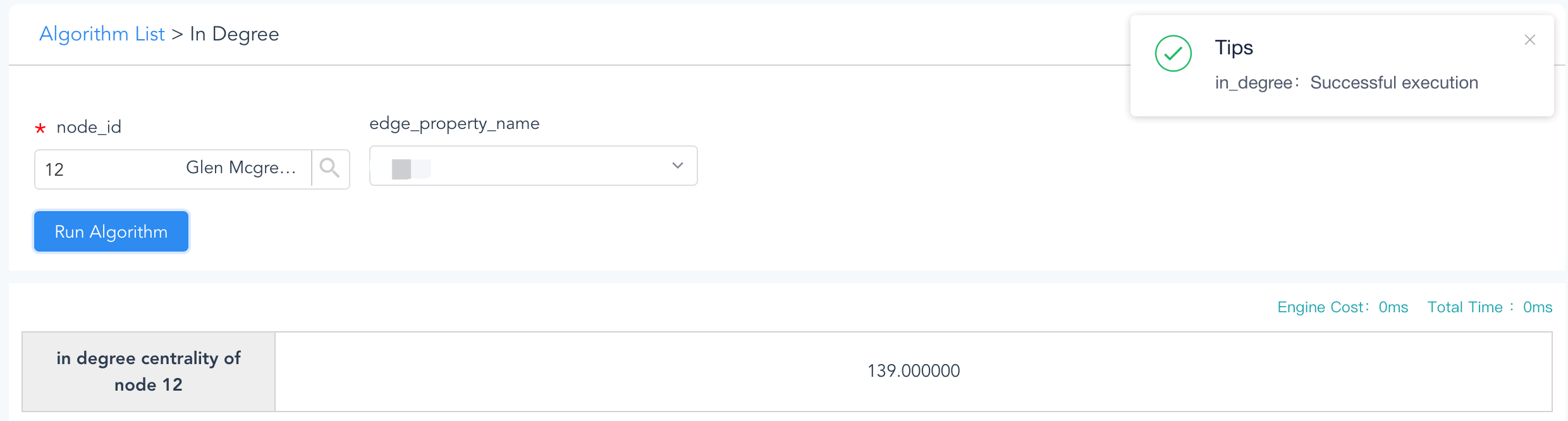Figure: Running Real-Time Algorithm - In Degree

Example 2: Calculate the In Degree with edge property 'rank' of node (_id = 12)

``````algo(in_degree)
.params( {node_id: 12, edge_property_name: "rank"} )
``````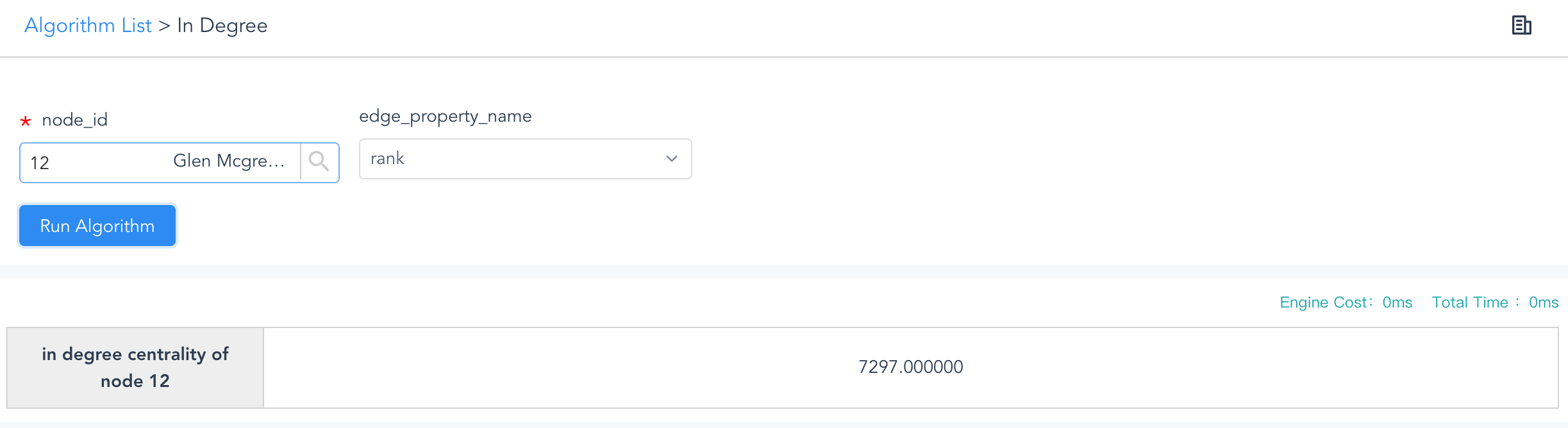Figure: In Degree by Edge Property

### `algo(degree)`

Basic     Real-time

Degree is the summed total of In Degree and Out Degree of node.

Configuration items for degree operations:

Item Data Type Specification Description
`<node_id>` int Ultipa ID To calculate for a specific node
`<edge_property_name>` string Edge property (numeric type) (Optional) The edge property (must be LTE first) to be used as the weight factor of edge, or use 1 as weight factor if not configured

Validity of `write_back()`:

Not supported.

Example 1: Calculate the degree of node id=12

``````algo(degree)
.params( {node_id: 12} )
``````

Example 2: Calculate the degree of node id=12 using edge property 'rank' as weight factor

``````algo(degree)
.params( {node_id: 12, edge_property_name: "rank"} )
``````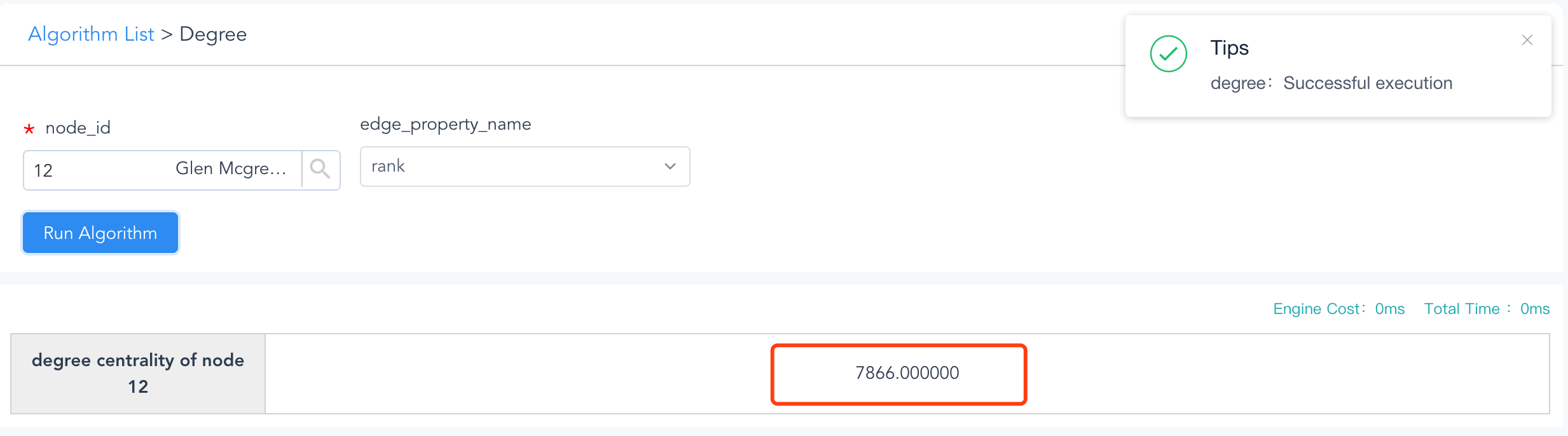Figure: Real-Time Algorithm - Degree with Edge Property

The above result is also verified by the results from previous examples.

### `algo(out_degree_all)`

Basic

Out Degree All is a degree calculation algorithm on out-degrees (weighted or not) for multiple nodes, or all nodes, in the graphset, the results can be ordered and partially returned.

Configuration items for whole-graph out degree operations:

Item Data Type Specification Description
`<ids>` []int Ultipa ID (Optional) To calculate for a group of nodes, or calculate for all nodes if not configured
`<edge_property_name>` string Edge property (numeric type) (Optional) The edge property (must be LTE first) to be used as the weight factor of edge, or use 1 as weight factor if not configured
`<limit>` int >0; -1 The maximum number of results to return; -1: return all the results
`<order>` string 'ASC' or 'DESC' (Optional) To arrange the results in ascending or descending order, or leave them un-ordered if not configured

Validity of `write_back()`:

Approach Destination
To database node property `#out_degree_all`
To disk file /

Example 1: Calculate the out degrees for nodes (id=1,2,3,4,5,6,7), arrange the results in descending order and return the top 3.

``````algo(out_degree_all)
.params( {ids: [1,2,3,4,5,6,7], limit: 3, order: 'DESC'} )
``````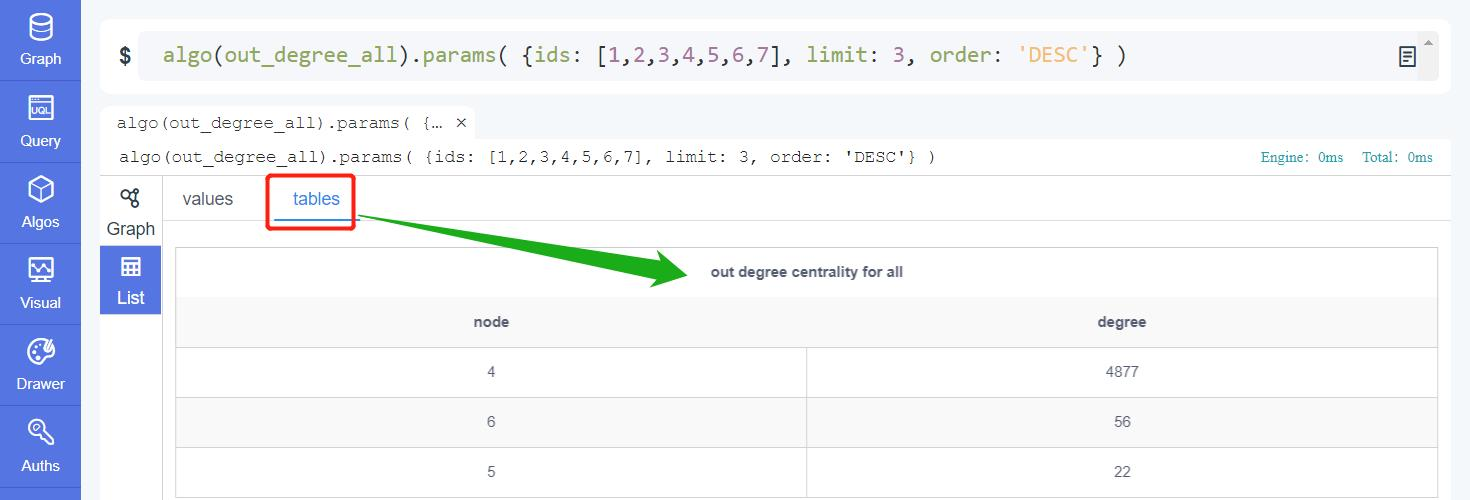Figure: Real-Time Algorithm - Out Degree All Top 3

Example 2: Calculate the whole graph out degrees with edge property 'rank' being the weight factor, and write back to the database.

``````algo(out_degree_all)
.params( {edge_property_name: "rank", limit: -1} )
.write_back()
``````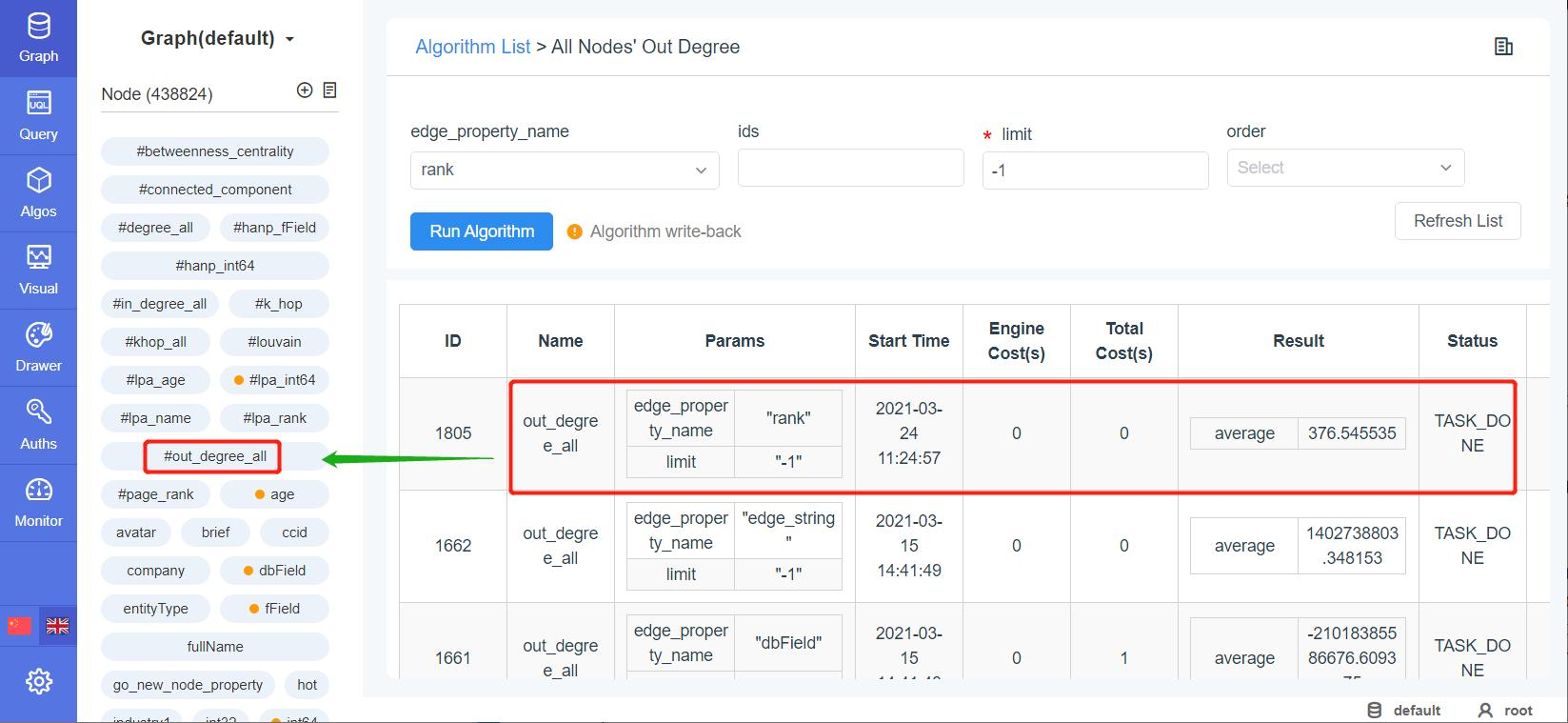Figure: Algorithm Writing Back to Graphset

As seen from above, after running the graph-wide out-degrees with `write_back()` included, a new node property is created, which will simply be updated (refreshed) if this algorithm is invoked again.

### `algo(in_degree_all)`

Basic

Similar to Out Degree All, the In Degree All calculates the nodes in-degrees for selective nodes or all nodes.

Configuration items for whole-graph in degree operations:

Item Data Type Specification Description
`<ids>` []int Ultipa ID (Optional) To calculate for a group of nodes, or calculate for all nodes if not configured
`<edge_property_name>` string Edge property (numeric type) (Optional) The edge property (must be LTE first) to be used as the weight factor of edge, or use 1 as weight factor if not configured
`<limit>` int >0; -1 The maximum number of results to return; -1: return all the results
`<order>` string 'ASC' or 'DESC' (Optional) To arrange the results in ascending or descending order, or leave them un-ordered if not configured

Validity of `write_back()`:

Approach Destination
To database node property `#in_degree_all`
To disk file /

Example 1: Calculate In Degree for nodes (id=1,2,3,4,5,6,7), arrange the results in descending order and return the top 3.

``````algo(in_degree_all)
.params( {ids: [1,2,3,4,5,6,7], limit: 3, order: 'DESC'} )
``````

Example 2: Calculate all nodes' In Degree for node property 'rank'

``````algo(in_degree_all)
.params( {edge_property_name: "rank", limit: -1} )
``````

### `algo(degree_all)`

Basic

Degree All is the summed total of In Degree All and Out Degree All that applied to selective nodes or graph-wide all nodes.

Configuration items for whole-graph degree operations:

Item Data Type Specification Description
`<ids>` []int Ultipa ID (Optional) To calculate for a group of nodes, or calculate for all nodes if not configured
`<edge_property_name>` string Edge property (numeric type) (Optional) The edge property (must be LTE first) to be used as the weight factor of edge, or use 1 as weight factor if not configured
`<limit>` int >0; -1 The maximum number of results to return; -1: return all the results
`<order>` string 'ASC' or 'DESC' (Optional) To arrange the results in ascending or descending order, or leave them un-ordered if not configured

Validity of `write_back()`:

Approach Destination
To database node property `#degree_all`
To disk file /

Example 1: Calculate degrees for nodes (id=1,2,3,4,5,6,7), arrange the results in descending order and return the top 3.

``````algo(degree_all)
.params( {ids: [1,2,3,4,5,6,7], limit: 3, order: 'DESC'} )
``````

Example 2: Calculate all nodes' degree based on edge property 'rank'

``````algo(degree_all)
.params( {edge_property_name: "rank", limit: -1} )
``````

## Centrality

### `algo(closeness)`

Closeness centrality (or closeness) of a node is the reciprocal of sum of its distances (length of the shortest path) to all the other nodes within its connected component in the graphset. By this definition, the more central a node is in the graph, the closer it is to the other nodes, the samller the aforementioned sum of distances, and the bigger the reciprocal.

Closeness was first defined by American psychosociologist Alex Bavelas in 1950 as the reciprocal of the farness: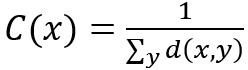Obviously, the formula above would make the eventual C(x) too small to be meaningful, and with Ultipa graph it is normalized by multiplying k-1, which stands for the number of nodes excluding the subject node, and C(x) will always carry a value from 0 to 1: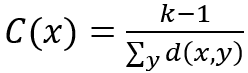Closeness centrality is a shortest-path-based algorithm that its computational complexity grows exponentially as the graphset gets larger. Ultipa Graph provides accurate closeness centrality calculation for graphsets with less than 10k nodes and edges. For cases of larger graphsets, the calculation is approximated by sampling, with a sample number which is the base-10 logarithm of total number of nodes `log(total_node_number)`.

Configuration items for closeness centrality operation:

Item Data Type Specification Description
`<ids>` []int Ultipa ID (Optional) To calculate for a group of nodes, or calculate for all nodes if not configured
`<limit>` int >0; -1 The maximum number of results to return; -1: return all the results

Validity of `write_back()`:

Not supported.

Example: Calculate the closeness of nodes (_id=1,2,3)

``````algo(closeness).params({ ids: [1,2,3], limit: -1 })
``````

Run this example to a graph with below structure: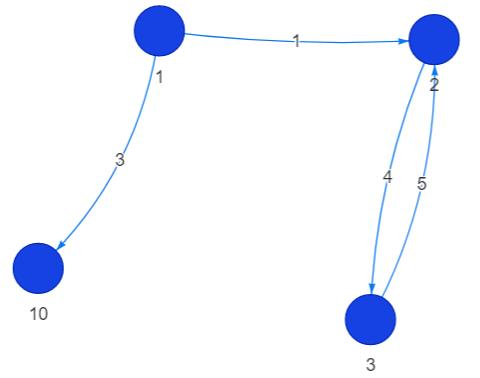Figure: Running Real-Time Algorithm – Closeness Centrality

And acquire below result: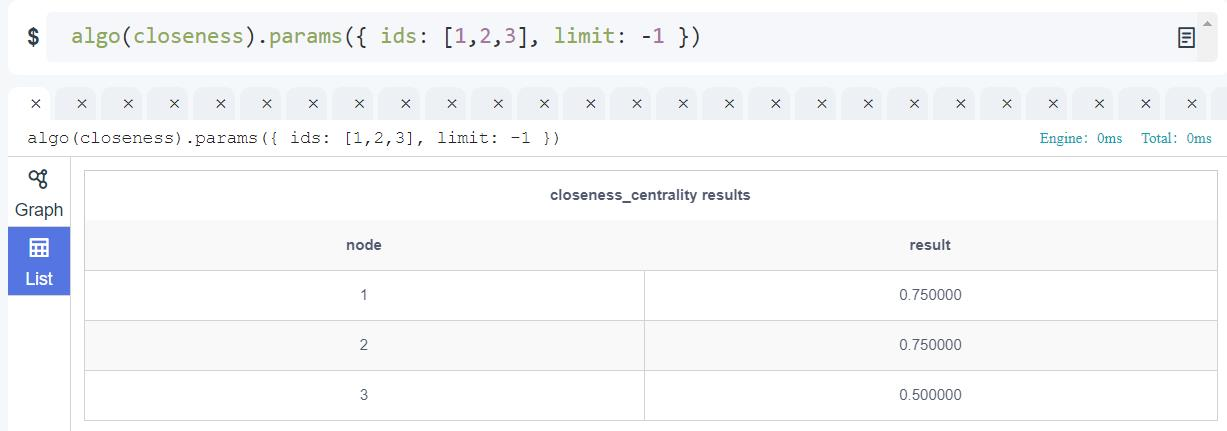Figure: Running Real-Time Algorithm – Closeness Centrality

### `algo(out_closeness)`

Out-Closeness Centrality is out-degree closeness centrality. The difference between closeness and out-closeness is that during the shortest-path calculation, out-closeness only calculates paths form by out-degree (right-directing) edges.

Configuration items for out-degree closeness centrality operation:

Item Data Type Specification Description
`<ids>` []int Ultipa ID (Optional) To calculate forf a group of nodes, or calculate for all nodes if not configured
`<limit>` int >0; -1 The maximum number of results to return; -1: return all the results

Validity of `write_back()`:

Not supported.

Example: Calculate out-closeness of nodes (_id=1,2,3)

``````algo(out_closeness).params({ ids: [1,2,3], limit: -1 })
``````

Run this example to the previous graph and get below result: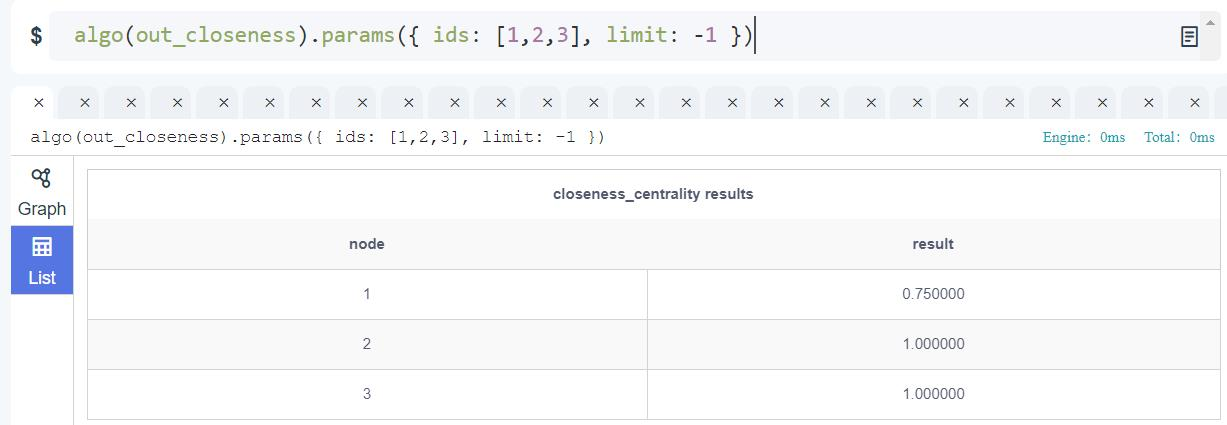Figure: Out Closeness for a Certain Node

### `algo(in_closeness)`

In Closeness Centrality is short for In-degree Close Centrality, similar to closeness centrality except that it only calculates in-degree-edge (left-directing) formed paths from the subject node.

Configuration items for in-degree closeness centrality operation:

Item Data Type Specification Description
`<ids>` []int Ultipa ID (Optional) To calculate for a group of nodes, or calculate for all nodes if not configured
`<limit>` int >0; -1 The maximum number of results to return; -1: return all the results

Validity of `write_back()`:

Not supported.

Example: Calculate the In-closeness of nodes id=(_id=1,2,3)

``````algo(in_closeness).params({ ids: [1,2,3], limit: -1 })
``````

Run this example to the previous graph and get below result: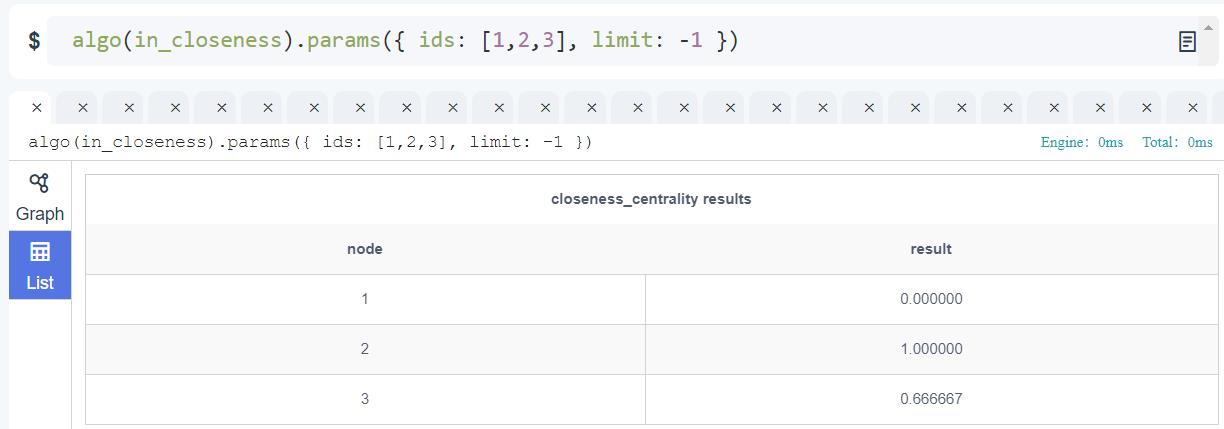Figure: In_Closeness_Centrality for a Specific Node

### `algo(graph_centrality)`

Graph centrality of a node is the reciprocal of its longest distance (length of the shortest-path) to all the other nodes within the connected component. It also produces a value between 0 and 1, and can be expressed as below: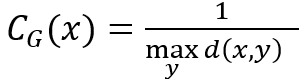In network analysis, graph centrality is used to identify the most important node in the graph, such as the most influential persons in a social network, the super-spreaders of CoVID-19, etc.

Configuration items for graph centrality operation:

Item Data Type Specification Description
`<node_id>` int Ultipa ID To calculate for a specific node

Validity of `write_back()`:

Not supported.

Example: Calculate graph centrality of node _id=1

``````algo(graph_centrality).params({ node_id: 1 })
``````

Run this example to the previous graph and get below result: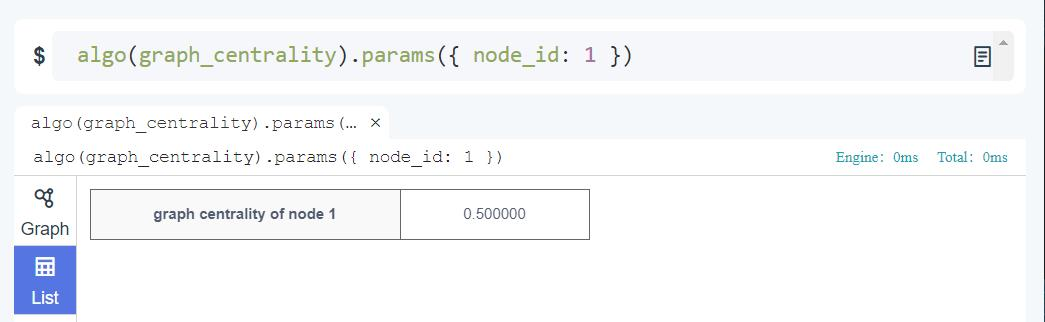Figure: Centrality Calculation for a Node (by _id)

Note that the graph centrality works against the entire connected component, the example above has a graph that has only 1 connected component, therefore the computational complexity is equivalent to finding out the largest K-hop of the subject node and inverse it. In the above example, the largest K-hop for node 1 is 2-hop (namely, any queries greater than 2-hop will get zero result), therefore 1/2=0.5 is the graph centrality for node 1.

### `algo(betweenness_centrality)`

Betweenness centrality of a node represents how frequently the node appears in the shortest paths between each pair of other nodes in its connected component. Betweenness centrality of a node x is given by the below expression: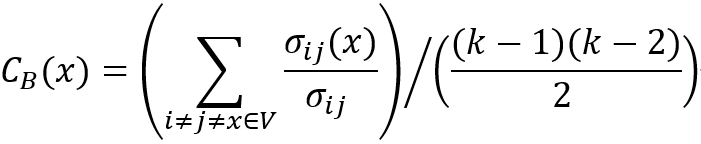Where the denominator σ is the total number of shortest paths from node i to node j, and the numerator σ(x) is the number of those paths passing through node x. The summation of σ(x)/σ is normalized by dividing (k-1)(k-2)/2, which is the combination number of node pairs. Obviously, betweenness centrality is a value from 0 to 1.

Betweenness centrality finds wide applications in network theory, for instance, in a telco-network, a node with high(er) betweenness centrality would have more control over the network.

In highly connected graphs, betweenness centrality against the entire graph tends to consume lots of computational resources and is very time-consuming, especially on graphs larger than 100K nodes and edges. Betweenness centrality by Ultipa Graph is approximated by sampling, with a sample number which is the base-10 logarithm of total number of nodes `log(total_node_number)`. Fluctuation is observable in the calculation result of a same subject node and will decrease as the graphset grows larger.

In case you need to do an accurate calculation of betweenness centrality, contact our team.

Configuration item for betweenness centrality operation:

Item Data Type Specification Description
`<limit>` int >0; -1 The maximum number of results to return; -1: return all the results

Validity of `write_back()`:

Approach Destination
To database node property `#betweenness_centrality`
To disk file /

Example1: Calculate graph-wide betweenness centrality

``````algo(betweenness_centrality)
.params({ limit: -1 })
.write_back()
``````

Run this example against a graphset with over 400k nodes and 3-million edges, and check the written-back results in the node property `#betweenness_centrality`: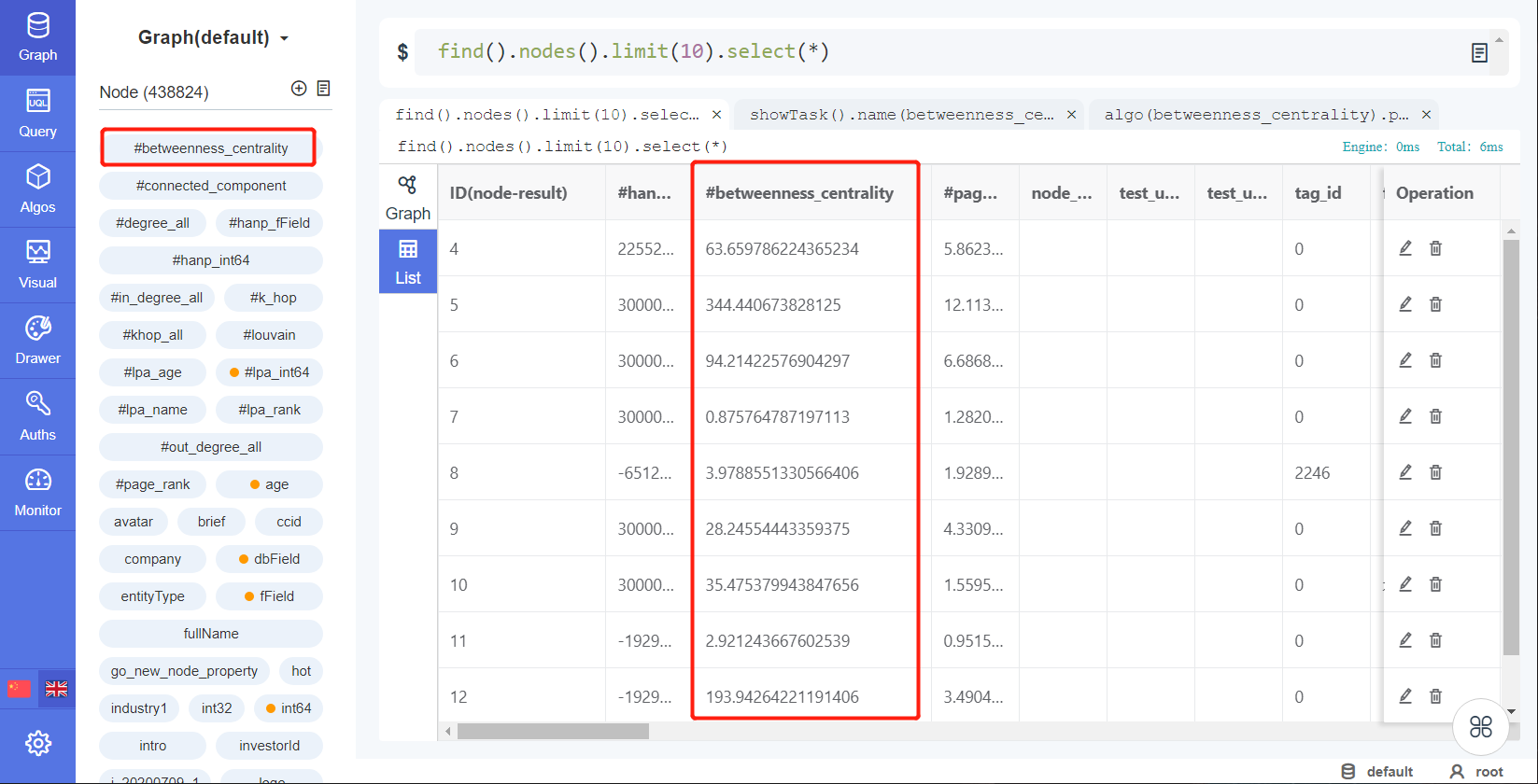Figure: Betweenness Centrality Writes Back